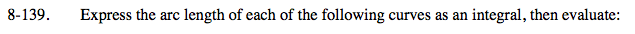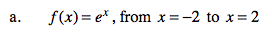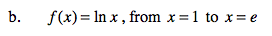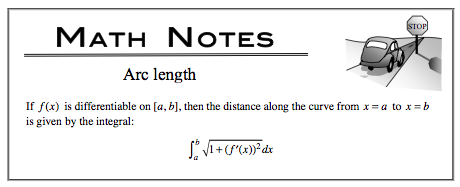### Home > CALC > Chapter 8 > Lesson 8.4.1 > Problem8-139

8-139.
1. Express the arc length of each of the following curves as an integral, then evaluate: Homework Help ✎

1. f(x) = ex , from x = –2 to x = 2

2. f(x) = ln x, from x = 1 to x = e